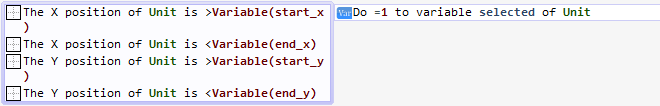# stratergy box select

i have my box working on click n drag of mouse but cant select any units.

." On the end of the draw box thing have every friendly unit check if its x > boxes leftmost part, x < boxes rightmost part, y > than its uppermost part an y < than its lowermost part, if all four are true, set unit to selected. "

but if someone could screenshot an exampe how todo this or explain exactly what this means would help alot

It is exactly what it means, maybe you don’t know how to get such values to test…
What is the box you are talking about, are you drawing it from the click positions with a shape painter object?

The idea is to get the start and end point: start_x, start_y, end_x, end_y
Then you have to test if the object position is inside this rectangle:This will work well only when the start position is the top left corner of the rectangle, and the end position is the bottom right corner, so maybe you’ll need to test the max and min:
min_x = min(start_x, end_x), min_y = min(start_y, end_y)
max_x = max(start_x, end_x), max_y = max(start_y, end_y)
Now min_x is the left position (no matter what is the start/end position), min_y is the top one, and so on1 Like